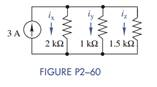### Create an Account

Already have account?

### Forgot Your Password ?

Home / Questions / Use current division in Figure P2 60 to find ix iy and iz Then show that the sum of these ...

# Use current division in Figure P2 60 to find ix iy and iz Then show that the sum of these currents equals the source current

Use current division in Figure P2−60 to find ix, iy, and iz. Then show that the sum of these currents equals the source current.Jun 15 2020 View more View Less

#### Answer (Solved)Subscribe To Get Solution